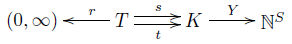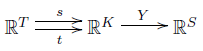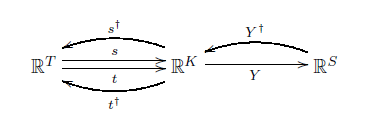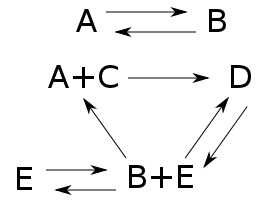# The Azimuth Project Blog - network theory (part 22)

This page is a blog article in progress, written by John Baez. To see discussions of this article as it was being written, go to the Azimuth Forum. To see the final polished version, visit the Azimuth Blog.

Okay, now let’s dig deeper into the proof of the deficiency zero theorem. We’re only going to prove a baby version, at first. Later we can enhance it:

Deficiency Zero Theorem (Baby Version). Suppose we have a weakly reversible reaction network with deficiency zero. Then for any choice of rate constants there exists an equilibrium solution of the rate equation where all species are present in nonzero amounts.

The first step is to write down the rate equation in a new, more conceptual way. It’s incredibly cool. You’ve probably seen Schrödinger’s equation, which describes the motion of a quantum particle:

$\displaystyle { \frac{d \psi}{d t} = -i H \psi }$

If you’ve been following this series, you’ve probably also seen the master equation, which describes the motion of a ‘stochastic’ particle:

$\displaystyle { \frac{d \psi}{d t} = H \psi }$

A ‘stochastic’ particle is one that’s carrying out a random walk, and now $\psi$ describes its probability to be somewhere, instead of its amplitude.

Today we’ll see that the rate equation for a reaction network looks somewhat similar:

$\displaystyle { \frac{d x}{d t} = Y H x^Y }$

where $Y$ is some matrix, and $x^Y$ is defined using a new thing called ‘matrix exponentiation’, which makes the equation nonlinear!

If you’re reading this you probably know how to multiply a vector by a matrix. But if you’re like me, you’ve never seen anyone take a vector and raise to the power of some matrix! I’ll explain it, don’t worry… right now I’m just trying to get you intrigued. It’s not complicated, but it’s exciting how this unusual operation shows up naturally in chemistry. That’s just what I’m looking for these days: new math ideas that show up in practical subjects like chemistry, and new ways that math can help people in these subjects.

Since we’re looking for an equilibrium solution of the rate equation, we actually want to solve

$\displaystyle { \frac{d x}{d t} = 0 }$

or in other words

$Y H x^Y = 0$

In fact we will do better: we will find a solution of

$H x^Y = 0$

And we’ll do this in two stages:

• First we’ll find all solutions of

$H \psi = 0$

This equation is linear, so it’s easy to understand.

• Then, among these solutions $\psi$, we’ll find one that also obeys

$\psi = x^Y$

This is a nonlinear problem involving matrix exponentiation, but still, we can do it, using a clever trick called ‘logarithms’.

Putting the pieces together, we get our solution of

$H x^Y = 0$

and thus our equilibrium solution of the rate equation:

$\displaystyle { \frac{d x}{d t} = Y H x^Y = 0 }$

That’s a rough outline of the plan. But now let’s get started, because the details are actually fascinating. Today I’ll just show you how to rewrite the rate equation in this new way.

### The rate equation

Remember how the rate equation goes. We start with a stochastic reaction network, meaning a little diagram like this:This contains quite a bit of information:

• a finite set $T$ of transitions,

• a finite set $K$ of complexes,

• a finite set $S$ of species,

• a map $r: T \to (0,\infty)$ giving a rate constant for each transition,

source and target maps $s,t : T \to K$ saying where each transition starts and ends,

• a map $Y : K \to \mathbb{N}^S$ saying how each complex is made of species.

Given all this, the rate equation says how the amount of each species changes with time. We describe these amounts with a vector $x \in [0,\infty)^S$. So, we want a differential equation filling in the question marks here:

$\displaystyle{ \frac{d x}{d t} = ??? }$

Now last time, we started by thinking of $K$ as a subset of $\mathbb{N}^S$, and thus of the vector space $\mathbb{R}^S$. Back then, we wrote the rate equation as follows:

$\displaystyle{ \frac{d x}{d t} = \sum_{\tau \in T} r(\tau) \; \left(t(\tau) - s(\tau)\right) \; x^{s(\tau)} }$

where vector exponentiation is defined by

$x^s = x_1^{s_1} \cdots x_k^{s_k}$

when $x$ and $s$ are vectors in $\mathbb{R}^S$.

However, we’ve now switched to thinking of our set of complexes $K$ as a set in its own right that is mapped into $\mathbb{N}^S$ by $Y$. This is good for lots of reasons, like defining the concept of ‘deficiency’, which we did last time. But it means the rate equation above doesn’t quite parse anymore! Things like $s(\tau)$ and $t(\tau)$ live in $K$; we need to explicitly convert them into elements of $\mathbb{R}^S$ using $Y$ for our equation to make sense!

So now we have to write the rate equation like this:

$\displaystyle{ \frac{d x}{d t} = Y \sum_{\tau \in T} r(\tau) \; \left(t(\tau) - s(\tau)\right) \; x^{Y s(\tau)} }$

This looks more ugly, but if you’ve got even one mathematical bone in your body, you can already see vague glimmerings of how we’ll rewrite this the way we want:

$\displaystyle { \frac{d x}{d t} = Y H x^Y }$

Here’s how.

First, we extend our maps $s, t$ and $Y$ to linear maps between vector spaces:Then, we put an inner product on the vector spaces $\mathbb{R}^T$, $\mathbb{R}^K$ and $\mathbb{R}^S$. For $\mathbb{R}^K$ we do this in the most obvious way, by letting the complexes be an orthonormal basis. So, given two complexes $\kappa, \kappa'$, we define their inner product by

$\langle \kappa, \kappa' \rangle = \delta_{\kappa, \kappa'}$

We do the same for $\mathbb{R}^S$. But for $\mathbb{R}^T$ we define the inner product in a more clever way involving the rate constants. If $\tau, \tau' \in T$ are two transitions, we define their inner product by:

$\langle \tau, \tau' \rangle = \frac{1}{r(\tau)} \delta_{\tau, \tau'}$

This will seem perfectly natural when we continue our study of circuits made of electrical resistors, and if you’re very clever you can already see it lurking in Part 16. But never mind.

Having put inner products on these three vector spaces, we can take the adjoints of the linear maps between them, to get linear maps going back the other way:These are defined in the usual way—though we’re using daggers here they way physicists do, where mathematicians would prefer to see stars! For example, $s^\dagger : \mathbb{R}^K \to \mathbb{R}^T$ is defined by the relation

$\langle s^\dagger \phi, \psi \rangle = \langle \phi, s \psi \rangle$

and so on.

Next, we set up a random walk on the set of complexes. Remember, our reaction network is a graph with complexes as vertices and transitions as edges, like this:Each transition $\tau$ has a number attached to it: the rate constant $r(\tau)$. So, we can randomly hop from complex to complex along these transitions, with probabilities per unit time described by these numbers. The probability of being at some particular complex will then be described by a function

$\psi : K \to \mathbb{R}$

which also depends on time, and changes according to the equation

$\displaystyle { \frac{d \psi}{d t} = H \psi }$

for some Hamiltonian

$H : \mathbb{R}^K \to \mathbb{R}^K$

I defined this Hamiltonian back in Part 15, but now I see a slicker way to write it:

$H = (t - s) s^\dagger$

I’ll justify this next time. For now, the main point is that with this Hamiltonian, the rate equation is equivalent to this:

$\displaystyle{ \frac{d x}{d t} = Y H x^Y }$

The only thing I haven’t defined yet is the funny exponential $x^Y$. That’s what makes the equation nonlinear. We’re taking a vector to the power of a matrix and getting a vector. This sounds weird—but it actually makes sense!

It only makes sense because we have chosen bases for our vector spaces. To understand it, let’s number our species $1, \dots, k$ as we’ve been doing all along, and number our complexes $1, \dots, \ell$. Our linear map $Y : \mathbb{R}^K \to \mathbb{R}^S$ then becomes a $k \times \ell$ matrix of natural numbers. Its entries say how many times each species shows up in each complex:

$Y = \left( \begin{array}{cccc} Y_{11} & Y_{12} & \cdots & Y_{1 \ell} \\ Y_{21} & Y_{22} & \cdots & Y_{2 \ell} \\ \vdots & \vdots & \ddots & \vdots \\ Y_{k1} & Y_{k2} & \cdots & Y_{k \ell} \end{array} \right)$

The entry $Y_{i j}$ says how many times the $i$th species shows up in the $j$th complex.

Now, let’s be a bit daring and think of the vector $x \in \mathbb{R}^S$ as a row vector with $k$ entries:

$x = \left(x_1 , x_2 , \dots , x_k \right)$

Then we can multiply $x$ on the right by the matrix $Y$ and get a vector in $\mathbb{R}^K$:

$\begin{array}{ccl} x Y &=& \left( \begin{array}{cccc} x_1 , & x_2, &\dots, & x_k \end{array} \right) \; \left( \begin{array}{cccc} Y_{11} & Y_{12} & \cdots & Y_{1 \ell} \\ Y_{21} & Y_{22} & \cdots & Y_{2 \ell} \\ \vdots & \vdots & \ddots & \vdots \\ Y_{k1} & Y_{k2} & \cdots & Y_{k \ell} \end{array} \right) \\ \\ &=& \left( x_1 Y_{11} + \cdots + x_k Y_{k1}, \; \dots, \; x_1 Y_{1 \ell} + \cdots + x_k Y_{k \ell} \right) \end{array}$

So far, no big deal. But now you’re ready to see the definition of $x^Y$, which is very similar:

$\begin{array}{ccl} x^Y &=& {\left( \begin{array}{cccc} x_1 , & x_2 , & \dots, & x_k \end{array} \right)}^{ \left( \begin{array}{cccc} Y_{11} & Y_{12} & \cdots & Y_{1 \ell} \\ Y_{21} & Y_{22} & \cdots & Y_{2 \ell} \\ \vdots & \vdots & \ddots & \vdots \\ Y_{k1} & Y_{k2} & \cdots & Y_{k \ell} \end{array} \right)} \\ \\ &=& \left( x_1^{Y_{11}} \cdots x_k^{Y_{k1}} ,\; \dots, \; x_1^{Y_{1 \ell}} \cdots x_k^{Y_{k \ell}} \right) \end{array}$

It’s exactly the same, but with multiplication replacing addition, and exponentiation replacing multiplication! Apparently my class on matrices stopped too soon: we learned about matrix multiplication, but matrix exponentiation is also worthwhile.

What’s the point of it? Well, suppose you have a certain number of hydrogen molecules, a certain number of oxygen molecules, a certain number of water molecules, and so on—a certain number of things of each species. You can list these numbers and get a vector $x \in \mathbb{R}^S$. Then the components of $x^Y$ describe how many ways you can build up each complex from the things you have. For example,

$x_1^{Y_{11}} x_2^{Y_{21}} \cdots x_k^{Y_{k1}}$

say roughly how many ways you can build complex 1 by picking $Y_{11}$ things of species 1, $Y_{21}$ things of species 2, and so on.

Why ‘roughly’? Well, we’re pretending we can pick the same thing twice. So if we have 4 water molecules and we need to pick 3, this formula gives $4^3$. The right answer is $4 \times 3 \times 2$. To get this answer we’d need to use the ‘falling power’ $4^{\underline{3}} = 4 \times 3 \times 2$, as explained in Part 4. But the rate equation describes chemistry in the limit where we have lots of things of each species. In this limit, the ordinary power becomes a good approximation.

Theorem. The rate equation:

$\displaystyle{ \frac{d x}{d t} = Y \sum_{\tau \in T} r(\tau) \; \left(t(\tau) - s(\tau)\right) \; x^{Y s(\tau)} }$

is equivalent to this equation:

$\displaystyle{ \frac{d x}{d t} = Y (t - s) s^\dagger x^Y }$

or in other words:

$\displaystyle{ \frac{d x}{d t} = Y H x^Y }$

Proof. It’s enough to show

$\displaystyle{ (t - s) s^\dagger x^Y = \sum_{\tau \in T} r(\tau) \; \left(t(\tau) - s(\tau)\right) \; x^{Y s(\tau)} }$

So, we’ll compute $(t - s) s^\dagger x^Y$, and think about the meaning of each quantity we get en route.

We start with $x \in \mathbb{R}^S$. This is a list of numbers saying how many things of each species we have: our raw ingredients, as it were. Then we compute

$x^Y = (x_1^{Y_{11}} \cdots x_k^{Y_{k1}} , \dots, x_1^{Y_{1 \ell}} \cdots x_k^{Y_{k \ell}} )$

This is a vector in $\mathbb{R}^K$. It’s a list of numbers saying how many ways we can build each complex starting from our raw ingredients.

Alternatively, we can write this vector $x^Y$ as a sum over basis vectors:

$x^Y = \sum_{\kappa \in K} x_1^{Y_{1\kappa}} \cdots x_k^{Y_{k\kappa}} \; \kappa$

Next let’s apply $s^\dagger$ to this. We claim that

$\displaystyle{ s^\dagger \kappa = \sum_{\tau : s(\tau) = \kappa} r(\tau) \; \tau }$

In other words, we claim $s^\dagger \kappa$ is the sum of all the transitions having $\kappa$ as their source, weighted by their rate constants! To prove this claim, it’s enough to take the inner product of each side with any transition $\tau'$, and check that we get the same answer. For the left side we get

$\langle s^\dagger \kappa, \tau' \rangle = \langle \kappa, s(\tau') \rangle = \delta_{\kappa, s (\tau') }$

To compute the right side, we need to use the cleverly chosen inner product on $\mathbb{R}^T$. Here we get

$\displaystyle{ \left\langle \sum_{\tau : s(\tau) = \kappa} r(\tau) \tau, \; \tau' \right\rangle = \sum_{\tau : s(\tau) = \kappa} \delta_{\tau, \tau'} = \delta_{\kappa, s(\tau')} }$

In the first step here, the factor of $1 /r(\tau)$ in the cleverly chosen inner product canceled the visible factor of $r(\tau)$. For the second step, you just need to think for half a minute—or ten, depending on how much coffee you’ve had.

Either way, we we conclude that indeed

$\displaystyle{ s^\dagger \kappa = \sum_{\tau : s(\tau) = \kappa} r(\tau) \tau }$

Next let’s combine this with our formula for $x^Y$:

$x^Y = \sum_{\kappa \in K} x_1^{Y_{1\kappa}} \cdots x_k^{Y_{k\kappa}} \; \kappa$

We get this:

$s^\dagger x^Y = \sum_{\kappa, \tau : s(\tau) = \kappa} r(\tau) \; x_1^{Y_{1\kappa}} \cdots x_k^{Y_{k\kappa}} \; \tau$

In other words, $s^\dagger x^Y$ is a linear combination of transitions, where each one is weighted both by the rate it happens and how many ways it can happen starting with our raw ingredients.

Our goal is to compute $(t - s)s^\dagger x^Y$. We’re almost there. Remember, $s$ says which complex is the input of a given transition, and $t$ says which complex is the output. So, $(t - s) s^\dagger x^Y$ says the total rate at which complexes are created and/or destroyed starting with the species in $x$ as our raw ingredients.

That sounds good. But let’s just pedantically check that everything works. Applying $t - s$ to both sides of our last equation, we get

$\displaystyle{ (t - s) s^\dagger x^Y = \sum_{\kappa, \tau : s(\tau) = \kappa} r(\tau) \; x_1^{Y_{1\kappa}} \cdots x_k^{Y_{k\kappa}} \; \left( t(\tau) - s(\tau)\right) }$

Remember, our goal was to prove that this equals

$\displaystyle{ \sum_{\tau \in T} r(\tau) \; \left(t(\tau) - s(\tau)\right) \; x^{Y s(\tau)} }$

But if you stare at these a while and think, you’ll see they’re equal.   █

It took me a couple of weeks to really understand this, so I’ll be happy if it takes you just a few days. It seems peculiar at first but ultimately it all makes sense. The interesting subtlety is that we use the linear map called ‘multiplying by $Y$’:

$\begin{array}{ccc} \mathbb{R}^K &\to& \mathbb{R}^S \\ \psi &\mapsto& Y \psi \end{array}$

to take a bunch of complexes and work out the species they contain, while we use the nonlinear map called ‘raising to the $Y$th power’:

$\begin{array}{ccc} \mathbb{R}^S &\to& \mathbb{R}^K \\ x &\mapsto& x^Y \end{array}$

to take a bunch of species and work out how many ways we can build each complex from them. There is much more to say about this: for example, these maps arise from a pair of what category theorists call ‘adjoint functors’. But I’m worn out and you probably are too, if you’re still here at all.

### References

I found this thesis to be the most helpful reference when I was trying to understand the proof of the deficiency zero theorem:

• Jonathan M. Guberman, Mass Action Reaction Networks and the Deficiency Zero Theorem, B.A. thesis, Department of Mathematics, Harvard University, 2003.

I urge you to check it out. In particular, Section 3 and Appendix A discuss matrix exponentiation. Has anyone discussed this before?

Here’s another good modern treatment of the deficiency zero theorem:

• Jeremy Gunawardena, Chemical reaction network theory for in silico biologists, 2003.

The theorem was first proved here:

• Martin Feinberg, Chemical reaction network structure and the stability of complex isothermal reactors: I. The deficiency zero and deficiency one theorems, Chemical Engineering Science 42 (1987), 2229-2268.

However, Feinberg’s treatment here relies heavily on this paper:

• F. Horn and R. Jackson, General mass action kinetics, Archive for Rational Mechanics and Analysis 47 (1972), 81-116.

(Does anyone work on ‘irrational mechanics’?) These lectures are also very helpful:

• Martin Feinberg, Lectures on reaction networks, 1979.

If you’ve seen other proofs, let us know.

category: blog